### IMO Shortlist 2014 problem G7

Kvaliteta:
Avg: 0,0
Težina:
Avg: 9,0

Let$ABC$ be a triangle with circumcircle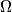$\Omega$ and incentre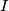$I$. Let the line passing through$I$ and perpendicular to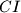$CI$ intersect the segment$BC$ and the arc$BC$ (not containing$A$) of$\Omega$ at points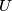$U$ and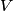$V$, respectively. Let the line passing through$U$ and parallel to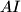$AI$ intersect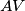$AV$ at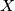$X$, and let the line passing through$V$ and parallel to$AI$ intersect$AB$ at$Y$. Let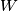$W$ and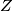$Z$ be the midpoints of$AX$ and$BC$, respectively. Prove that if the points$I$,$X$, and$Y$ are collinear, then the points$I$,$W$, and$Z$ are also collinear.

(U.S.A.)

Izvor: https://www.imo-official.org/problems/IMO2014SL.pdf